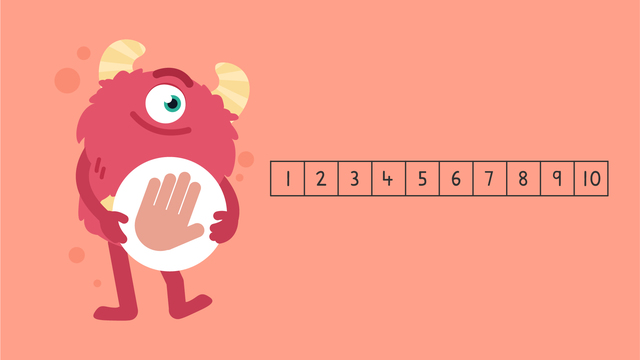# Counting to 10 (by ones)— Let's Practice!Rating

Ø 5.0 / 1 ratings
The authorsTeam Digital
Counting to 10 (by ones)— Let's Practice!
CCSS.MATH.CONTENT.K.CCA.1

## Basics on the topicCounting to 10 (by ones)— Let's Practice!

Let Razzi help you try counting to ten, and back, by ones!

### TranscriptCounting to 10 (by ones)— Let's Practice!

Razzi says get your thinking cap on because today we're going to practice, “Counting to Ten by Ones.” It's time to begin! Let's try counting forward from number one to number ten! We'll start at one and keep counting one more until we get to the number ten, let's go! One, two, three, four, five, six, seven, eight, nine, ten! Let's try it faster this time! One, two, three, four, five, six, seven, eight, nine, ten! Did you also get to ten each time? Let's count backward now! Are you ready... This time we'll start at ten and count back by one until we get to the number one, ready? Ten, nine, eight, seven, six, five, four, three, two, one! Let's try it faster now! Ten, nine, eight, seven, six, five, four, three, two, one! Did you also get to one each time? Razzi had so much fun practicing with you today! See you next time!

## Counting to 10 (by ones)— Let's Practice! exercise

Would you like to apply the knowledge you’ve learned? You can review and practice it with the tasks for the video Counting to 10 (by ones)— Let's Practice!.